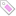#VLOOKUP查数据，有多个重复数据的方法，也可以用FILTER函数

VLOOKUP只能查找第一个值，如果要查找多个相同的值，可用辅助列的方式。

Tags: Excel

#XLOOKUP函数使用方法

=XLOOKUP(查找值,查找范围,结果范围,[容错值],[匹配方式],[查询模式])

=XLOOKUP(C1,A:A,B:B,"没找到") 在A列中查找等于C1的数据，显示B列对应的值，如果没有找到则显示“没找到”。

Tags: Excel

#AVERAGEIF函数AVERAGEIFS 多条件求平均数的使用方法

=Averageif（条件区域，条件，平均值区域）

=Averageifs（平均值区域，条件区域1，条件1，条件区域2，条件2）

Tags: Excel

#COUNTIF和COUNTIFS 多条件求和函数

COUNTIF函数语法:
=COUNTIF(条件区域,条件)

COUNTIFS:对满足多个条件的单元格计数
COUNTIFS（条件区域1，条件1，条件区域2，条件2..….条件区域n，条件n )

Tags: Excel

#SUMIF函数和SUMIFS函数使用方法

SUMIF函数通常用于对区域中符合指定的单个条件的值求和。

=SUMIF(条件区域,指定的条件,需要求和的区域)

=SUMIF(B:B,"1班",C:C)    对B列中等于"1班"对应的C列的值进行求和
=SUMIF(B:B,E1,C:C)        对B列中等于E1的数据对应的C列的值进行求和
=SUMIF(C:C,">100")        对C列中 大于100的数据进行求和
=SUMIF(C:C,"<"&AVERAGE(C:C))   对C列中小于平均数的值进行求和
=SUMIF(B:B,"*",C:C)  对B列不为空的单元格对应的C列的值进行求和

#Excel 向左匹配之 index+match

index + match：灵活性高

index(目标列,match(查找值,查找列,0))

- 0 ：精准匹配，从上往下能匹配到的第一个值
- 1 ：从上往下找<=查找值的最大值，查找区域必须升序排序

Tags: Excel

Tags: 身份证号码

#批量校验身份证号码是否正确excel代码

=IF(MID("10X98765432",MOD(SUMPRODUCT(MID(A2,ROW(\$1:\$17),1)*2^(18-ROW(\$1:\$17))),11)+1,1)=RIGHT(A2),"正确","错误")

=IF(LEN(A2)=18,RIGHT(A2,1)=MID("10X98765432",1+MOD(SUMPRODUCT(MID(LEFT(A2,17),ROW(\$1:\$17),1)*MOD(2^(18-ROW(\$1:\$17)),11)),11),1),FALSE)

Tags: Excel